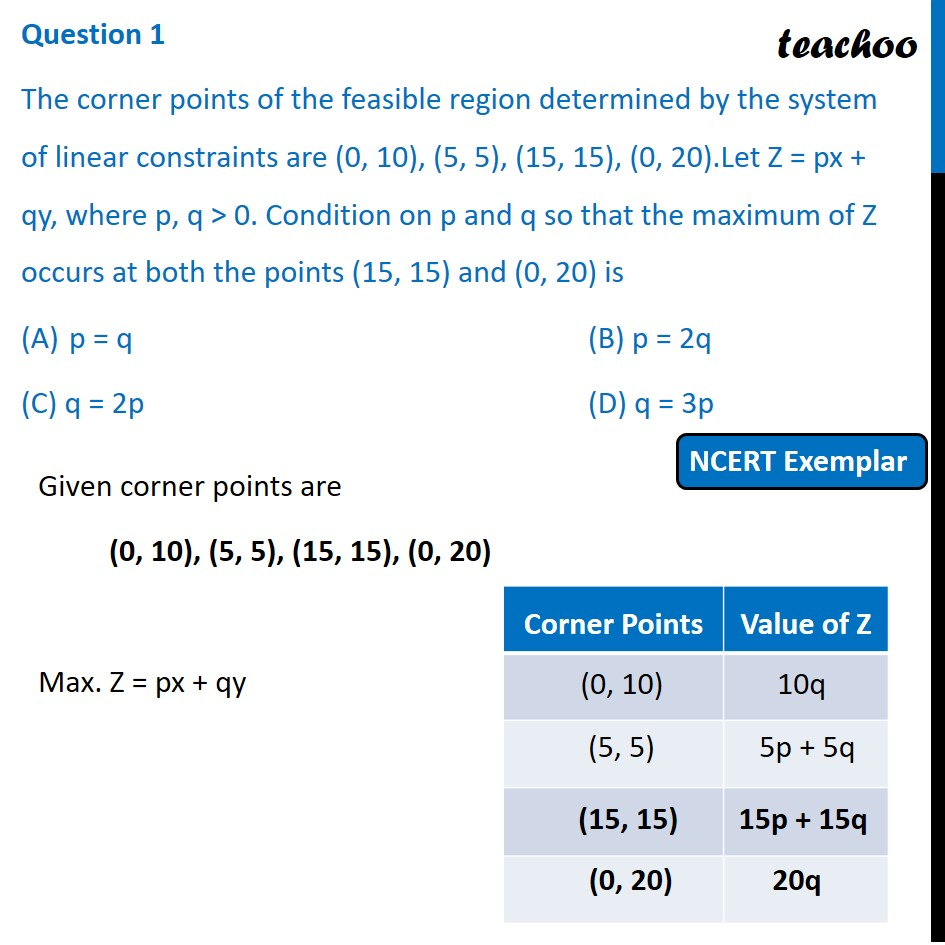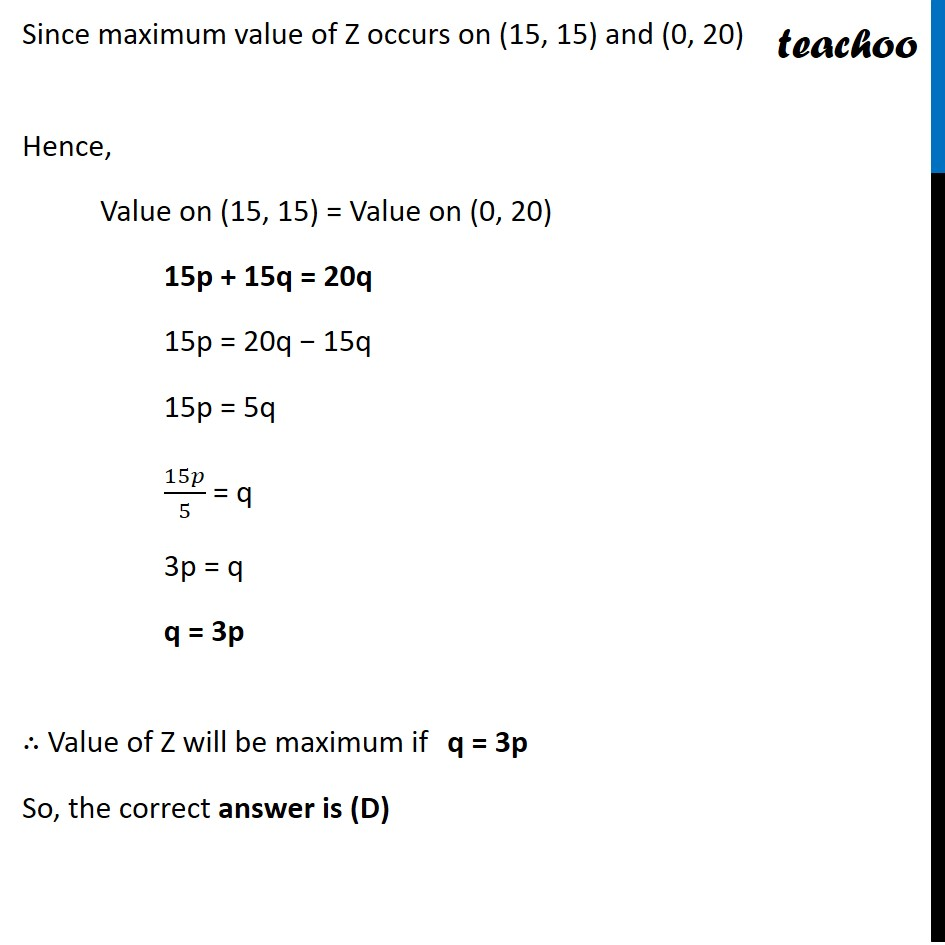NCERT Exemplar - MCQs

Chapter 12 Class 12 Linear Programming
Serial order wise

## (C) q = 2p               (D) q = 3p

This question is similar to Ex 12.2, 11 - Chapter 12 Class 12 - Linear ProgrammingLearn in your speed, with individual attention - Teachoo Maths 1-on-1 Class

### Transcript

Question 1 The corner points of the feasible region determined by the system of linear constraints are (0, 10), (5, 5), (15, 15), (0, 20).Let Z = px + qy, where p, q > 0. Condition on p and q so that the maximum of Z occurs at both the points (15, 15) and (0, 20) is p = q (B) p = 2q (C) q = 2p (D) q = 3p Given corner points are (0, 10), (5, 5), (15, 15), (0, 20) Max. Z = px + qy Since maximum value of Z occurs on (15, 15) and (0, 20) Hence, Value on (15, 15) = Value on (0, 20) 15p + 15q = 20q 15p = 20q − 15q 15p = 5q 15𝑝/5 = q 3p = q q = 3p ∴ Value of Z will be maximum if q = 3p So, the correct answer is (D)